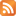# Math for Art class

I just finished teaching my Processing class at the Crucible.

The students were sharp and picked things up quickly, but I was surprised to find that some were unfamiliar with simple trig functions [sin() and cos()] to draw circular motion.

Well, if you are doing algorithmic art (or algorithms in general!) there's some insanely cool stuff that ether you have forgotten or more likely nobody taught you in the first place. The bad news is that it's math. The good news is that we can use Processing to do the heavy lifting, and concentrate on the concepts rather than the calculation!

So here's a first stab at a syllabus:

1. Exponentials
1. Trigonometry review
2. Sines and cosines for circles, ellipses and harmonic motion
3. Exponentials for natural decay
4. Euler's Theorem just because it totally rocks
5. introduction to complex numbers
2. Matrix Math (oh my!)
1. Intro: matrices are a lazy way to do algebra!
2. Vectors: Polar and rectangular positions in 2D and 3D space
3. Color spaces and how to convert between them
4. Matrix coordinate transformations (e.g. scaling, rotation)
5. Conformal mapping in the complex plane
3. Space and Surfaces
1. Intro: polygons and normals
2. Tessellations, triangulations, and Voronoi
3. Particle systems, flocking, and boids
4. Iteration and Recursion
1. Intro: iterative and recursive functions
2. Spirals, Fibonacci and the Golden Mean
3. Fractals and fractal dimension
4. Cellular automata
5. Flames and reaction-diffusion
5. Randomness
1. Intro: random() and distributions
2. 1D randomness: white noise, random walks, and Martingales
3. 2D randomness: Perlin noise and fractal landscapes
6. Curves
1. Intro: Curvature and calculus
2. Conic sections
3. Interpolation
4. Splines and Bezier curves
7. Signal and image processing
1. Intro: template matching
2. Filters, kernels, and smoothing
3. Gentle introduction to the frequency domain
4. CompressionRSS feed